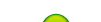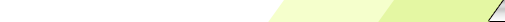Miércoles, 2023-10-04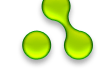My site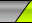Main | Registration | Login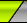Welcome Visitante | RSS
 Statistics Total en línea: 1 Invitados: 1 Usuarios: 0

# Update 2020: Anticovidian v.2 COVID-19: Hypothesis of the Lab Origin Versus a Zoonotic Event which can also be of a Lab Origin: https://zenodo.org/record/3988139

Appendixes from:

File Compression and Expansion of the Genetic Code by the use of the Yin/Yang Directions to find its Sphered Cube, by Fernando Castro-Chavez, J Biodivers Biopros Dev 1: 112. doi: 10.4172/2376-0214.1000112 [9 MB its PDF there, original site, slow download (there they are missing these Appendixes)]; Author's Zipped file: http://fdocc.ucoz.com/2/ijbbd.1000112.zip [4.05 MB] Alternative format by the NCBI of the NIH: https://www.ncbi.nlm.nih.gov/pmc/articles/PMC4203674

Appendix A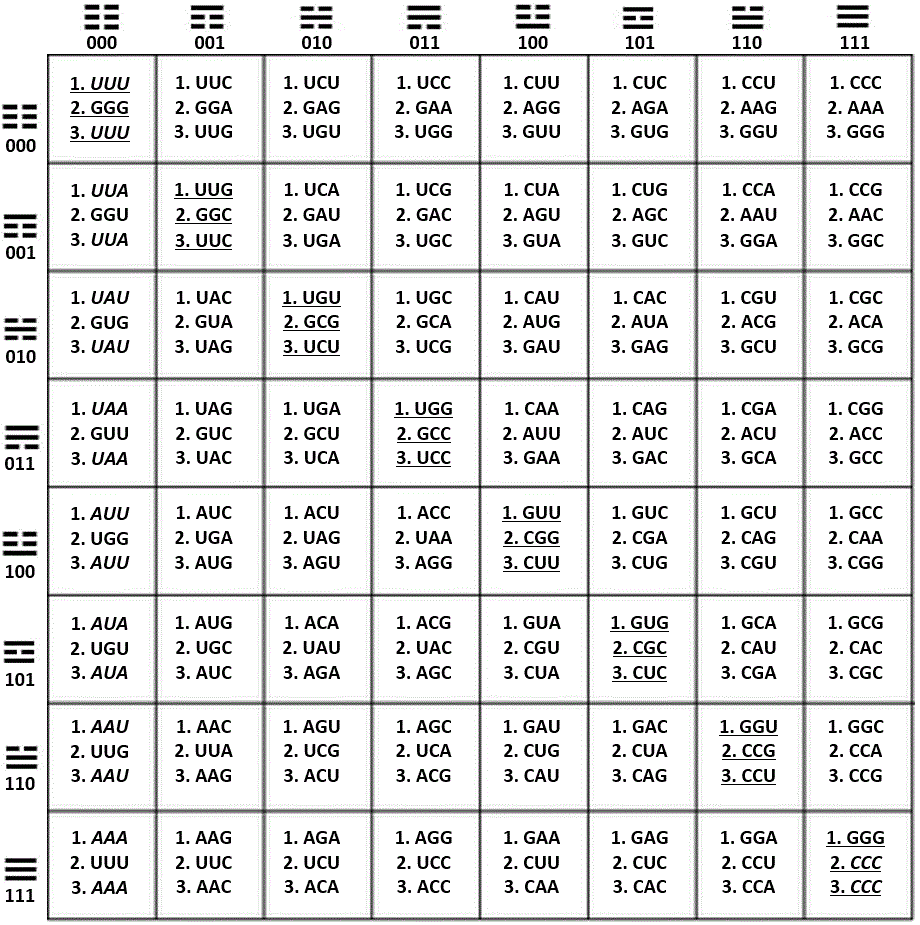Figure 28. Fusion of the three main sets of coordinates in one 64-grid table showing the trigrams that head the axis x and the axis y; within this table, the way the trigrams are read, is by locating first the trigram from the respective row (axis y), and above it the trigram located in the respective column (axis x). This set of two trigrams integrates the individual hexagram for each codon.

The three sets of normal, or standard, coordinates as presented in this article are:

1. H-bonds, axis x (A = U = 0, C = G = 1); Pur/Pyr ring, axis y (A = G = 0, C = U = 1).

2. Keto/Amino, axis x (G = U = 0, A = C = 1); Pur/Pyr ring, axis y (A = G = 0, C = U = 1).

3. H-bonds, axis x (A = U = 0, C = G = 1); Keto/Amino, axis y (G = U = 0, A = C = 1).

Appendix B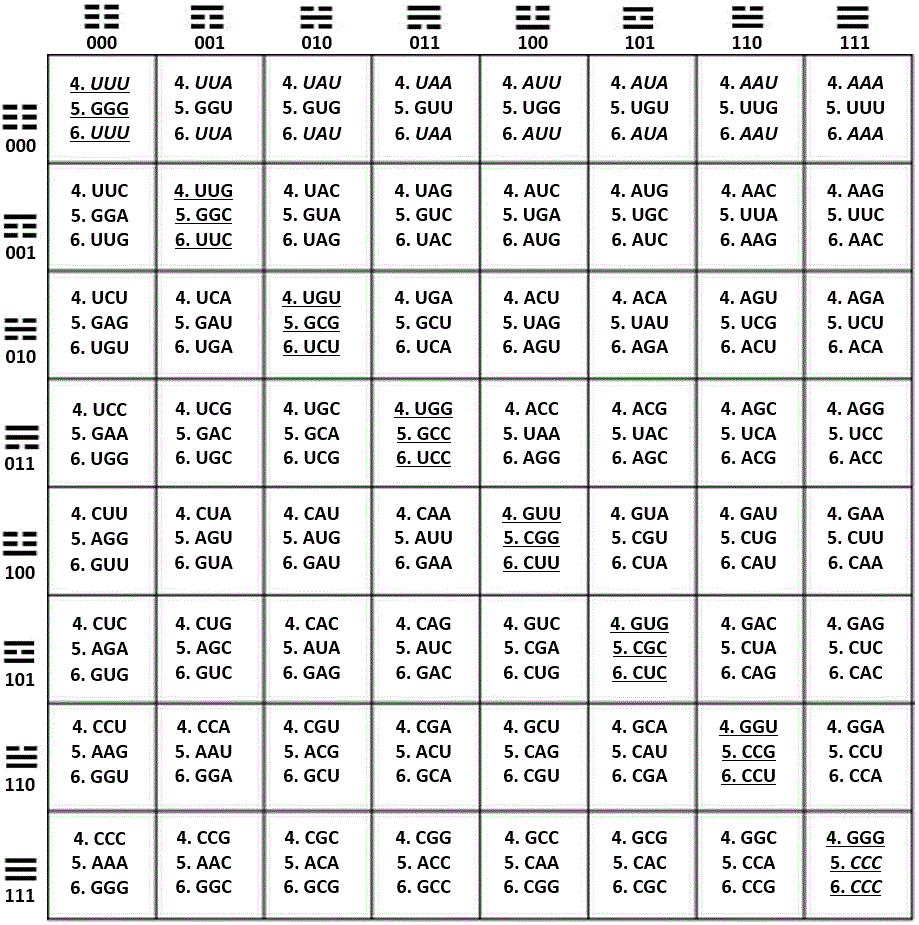Figure 29. Fusion of the three reciprocal sets of coordinates in one 64-grid table showing the trigrams that head the axis x and the axis y; within the table, the trigrams are read by locating first the trigram from the respective row (axis y), and above it, the trigram located in the respective column (axis x). This set of two trigrams integrates the individual hexagram for each codon.

The three sets of reciprocal, or mirror coordinates as presented in this article are the next ones:

4. Pur/Pyr ring, axis x (A = G = 0, C = U = 1), H-bonds, axis y (A = U = 0, C = G = 1).

5. Pur/Pyr ring, axis x (A = G = 0, C = U = 1), Keto/Amino, axis y (G = U = 0, A = C = 1).

6. Keto/Amino, axis x (G = U = 0, A = C = 1), H-bonds, axis y (A = U = 0, C = G = 1).

Notice that the only cells in common between App. A and App. B are the diagonal ones integrated by 1, 10, 19, 28, 37, 46, 55, 64 (underlined); in italics, the identities between the inner rows of codons.

Appendix C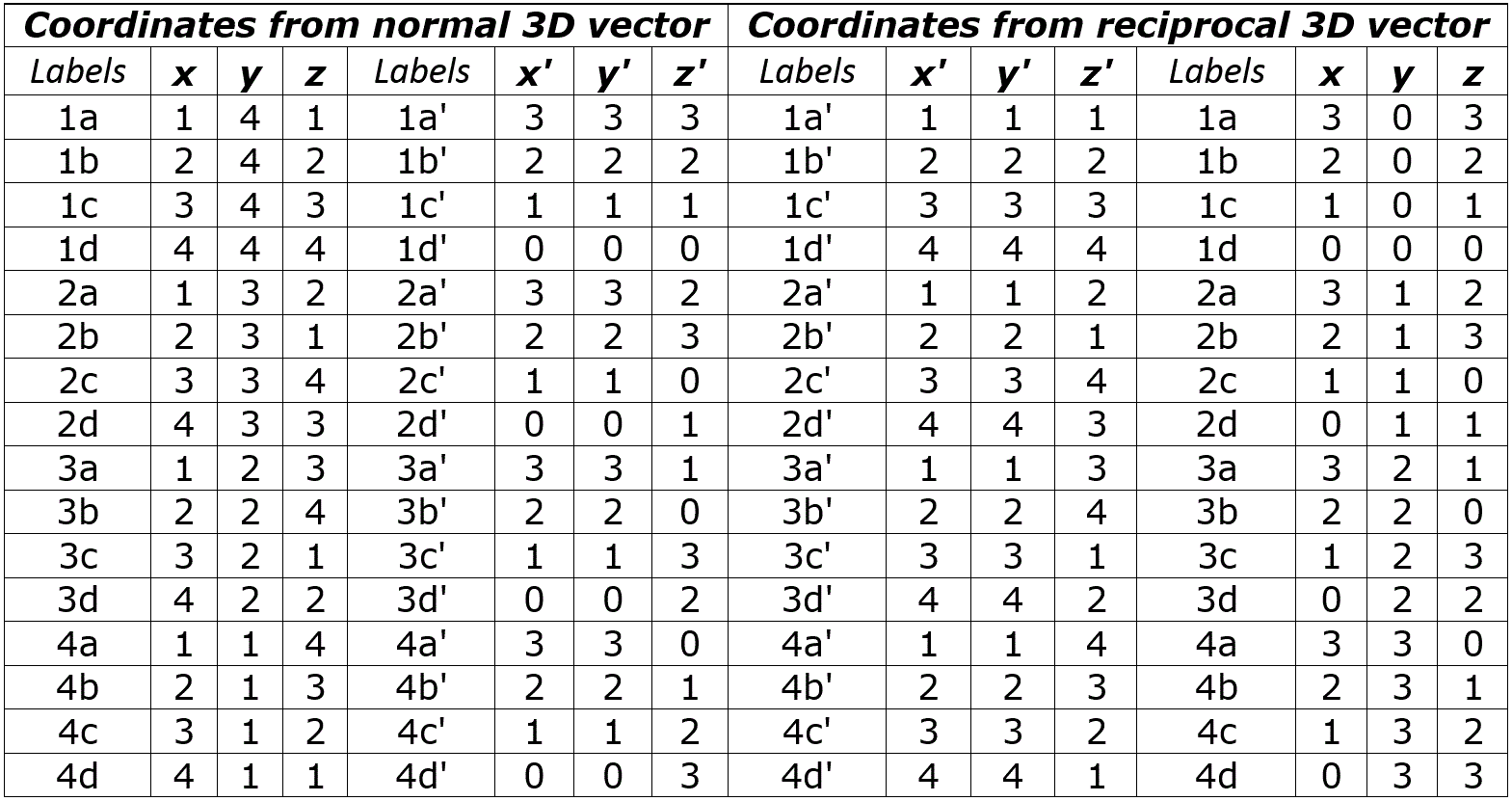Table 2. Coordinates to obtain the 3-D vector graphics of the Yin/Yang direction as they appear in the three main sets of Cartesian binary I Ching genetic code comparisons and their reciprocals; the coordinates presented in the left side are from the point of view of the normal side, while the right side looks at the coordinates from the point of view of the reciprocal side of the binary combinations of the codon properties discussed in this article.

Appendix D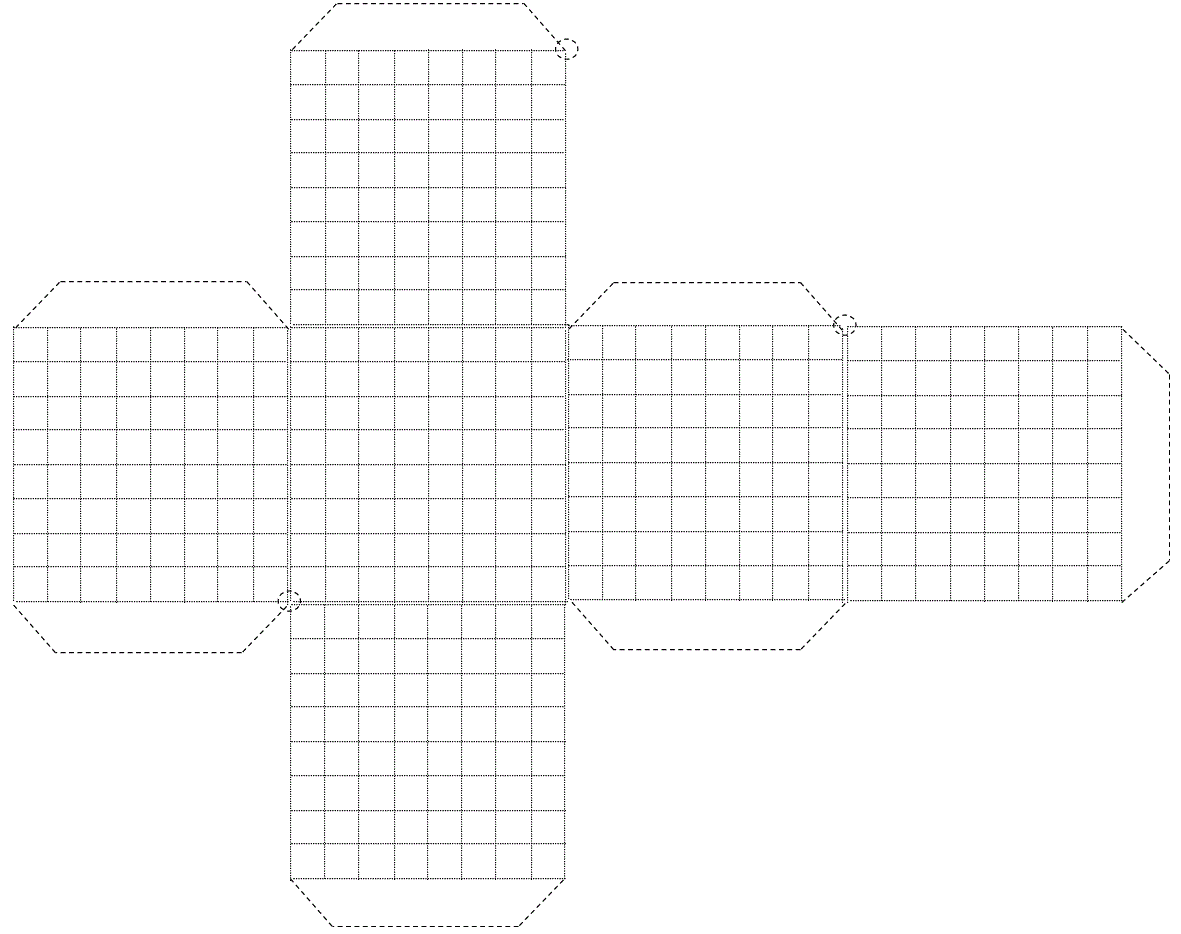Figure 30. Pattern to obtain the cube of 64x6=384 cells used in this article (the intermittent circles at the corners are the inclined axis of rotation).

These Appendixes have been saved at: http://liveweb.archive.org/web/20140617155918/http://fdocc.ucoz.com/index/0-122

//////////////////////

The full documentation of this original article as it was originally published can be found at:

Packaged supplement:

Personal communications with the reviewers:

Thanks!

Fernando Castro-Chavez.
 Search
 Site friends Create your own site